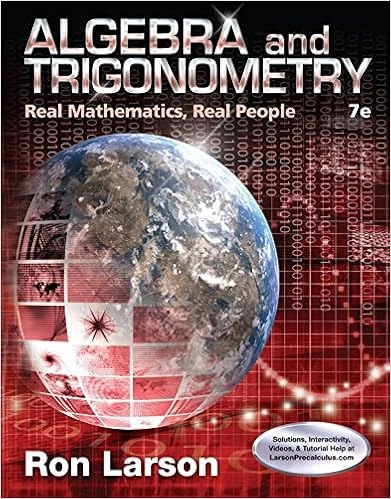# Linear Algebra with Applications (3rd Edition)

• Homework Help
• davidvictor
• 9
• 100% (2) 2 out of 2 people found this document helpful

This preview shows page 3 - 6 out of 9 pages.

##### We have textbook solutions for you!
The document you are viewing contains questions related to this textbook.The document you are viewing contains questions related to this textbook.
Chapter 4 / Exercise 63
Algebra and Trigonometry: Real Mathematics, Real People
LarsonExpert Verified
[2b.] The system ( A T A ) x = A T b is 3 6 6 18 x = 18 30 with (unique) solution 8 - 1 . This is the least square solution to the system Ax = b . [2c.] The vector p = 1 4 4 is in the column space of A . Moreover, the vector 0 - 1 1 spans the nullspace of P . Hence there are infinitely many choices for the vector q and they are q = p + t 0 - 1 1 , t R .
##### We have textbook solutions for you!
The document you are viewing contains questions related to this textbook.The document you are viewing contains questions related to this textbook.
Chapter 4 / Exercise 63
Algebra and Trigonometry: Real Mathematics, Real People
LarsonExpert Verified
3. [15 marks] 3a. [4 marks] Give a 3 × 3-matrix A with the following properties: i. A T = A - 1 . ii. det( A ) = 1. ( A is not allowed to be a diagonal matrix) 3b. [4 marks] Give a 3 × 3-matrix with the following properties: i. A T = A . ii. A 2 = A . iii. rk( A ) = 1. ( A is not allowed to be a diagonal matrix) 3c. [4 marks] Suppose A is a 5 × 3-matrix with orthonormal columns. Evaluate the following determinants: i det( A T A ) ii det( AA T ) iii det( A ( A T A ) - 1 A T ). 3d. [3 marks] Which value(s) of α R give det( A ) = 0, if A = α 2 3 - α α 0 3 2 5 ? Sol. [3a.] We consider, for example an orthogonal matrix A , with det( A ) = 1. Permutation matrices such as 0 1 0 0 0 1 1 0 0 or 0 0 1 1 0 0 0 1 0 have this property. [3b.] We may consider a projection matrix of rank 1: for example (cfr. question 2c.) A = v ( v T v ) - 1 v T , where v = 1 2 3 . Namely the matrix A = 1 14 1 2 3 2 4 6 3 6 9 . [3c.] det( A T A ) = det( I ) = 1 det( AA T ) = 0 det( A ( A T A ) - 1 A T ) = 0 AA T must have dependent columns and determinant zero because A ( A T x ) = 0 for any non-zero vector x in the nullspace of A T . The 3 × 5-matrix A T has 3 linearly independent (orthonormal!) rows and a non-trivial nullspace of dimension 5 - 3 = 2. Notice that det( A ( A T A ) - 1 A T ) = det( AA T ) = 0 as A T A = I . [3d.] det( A ) = 5 α ( α - 1) = 0. Therefore, α = 0 or α = 1.
4. [15 marks] Suppose the following information is known about a matrix A : i. A 2 0 0 = 2 4 - 6 ii. A 0 - 1 0 = - 2 - 4 6 iii. A is symmetric. The following questions refer to any matrix A with the above properties
•••